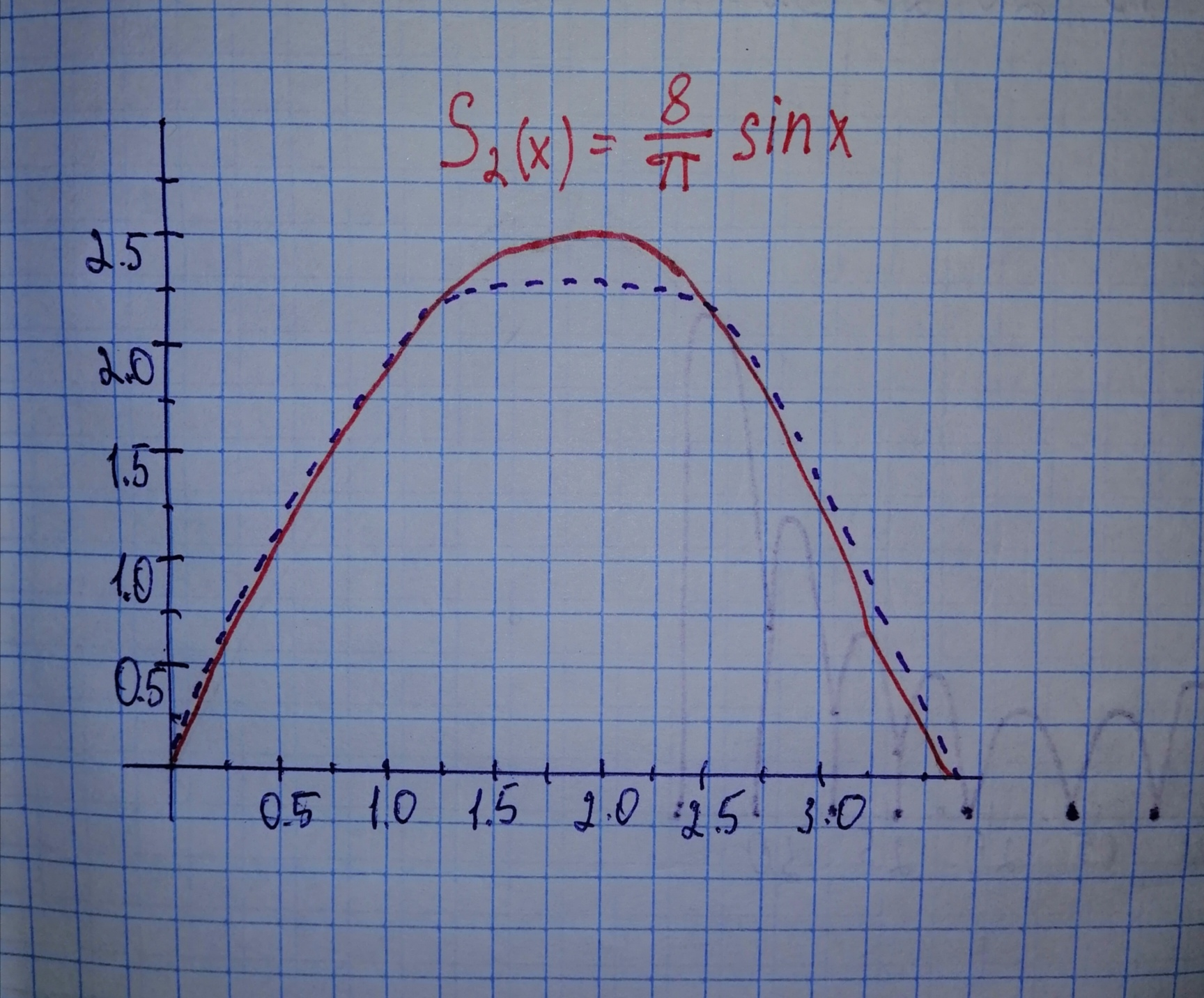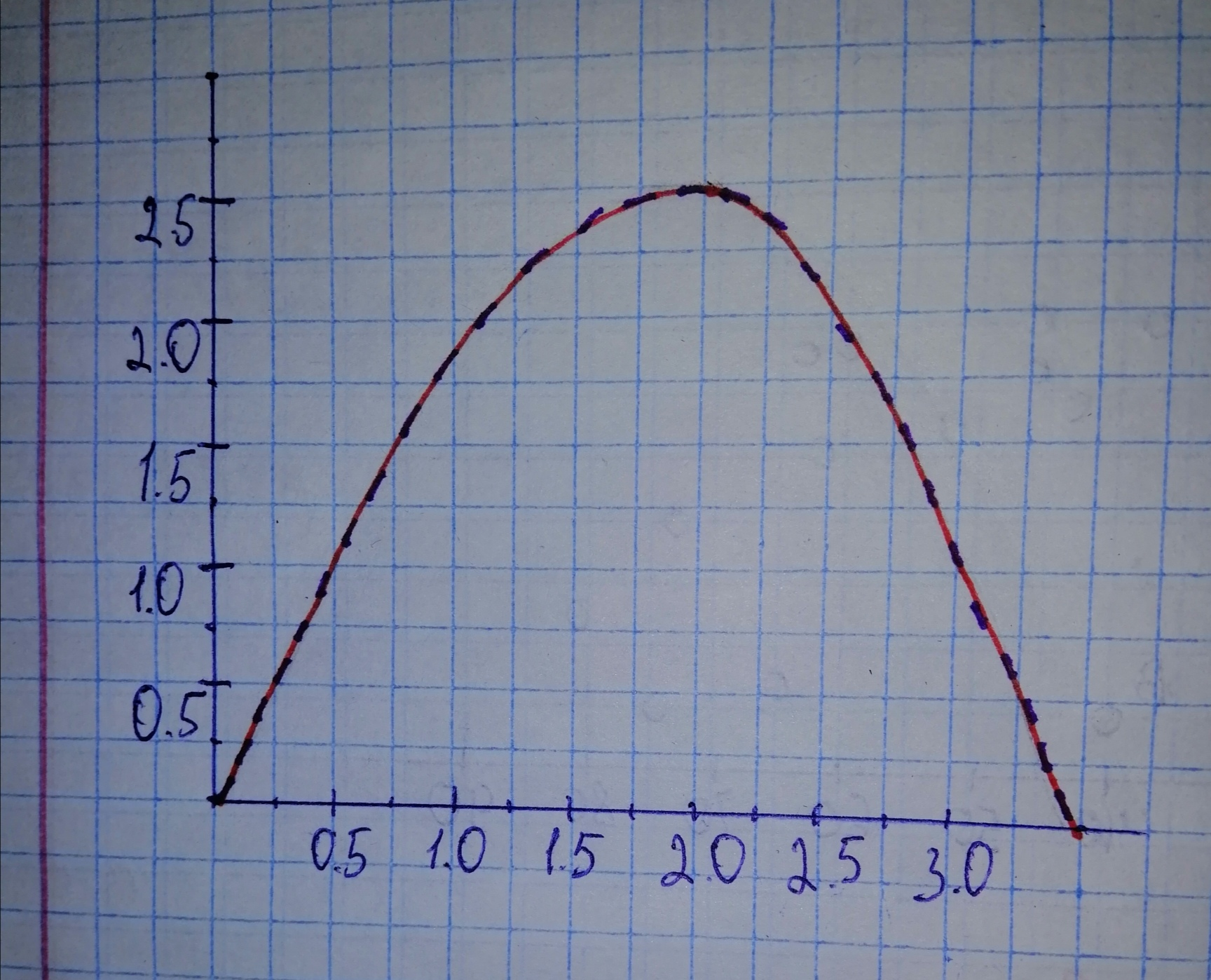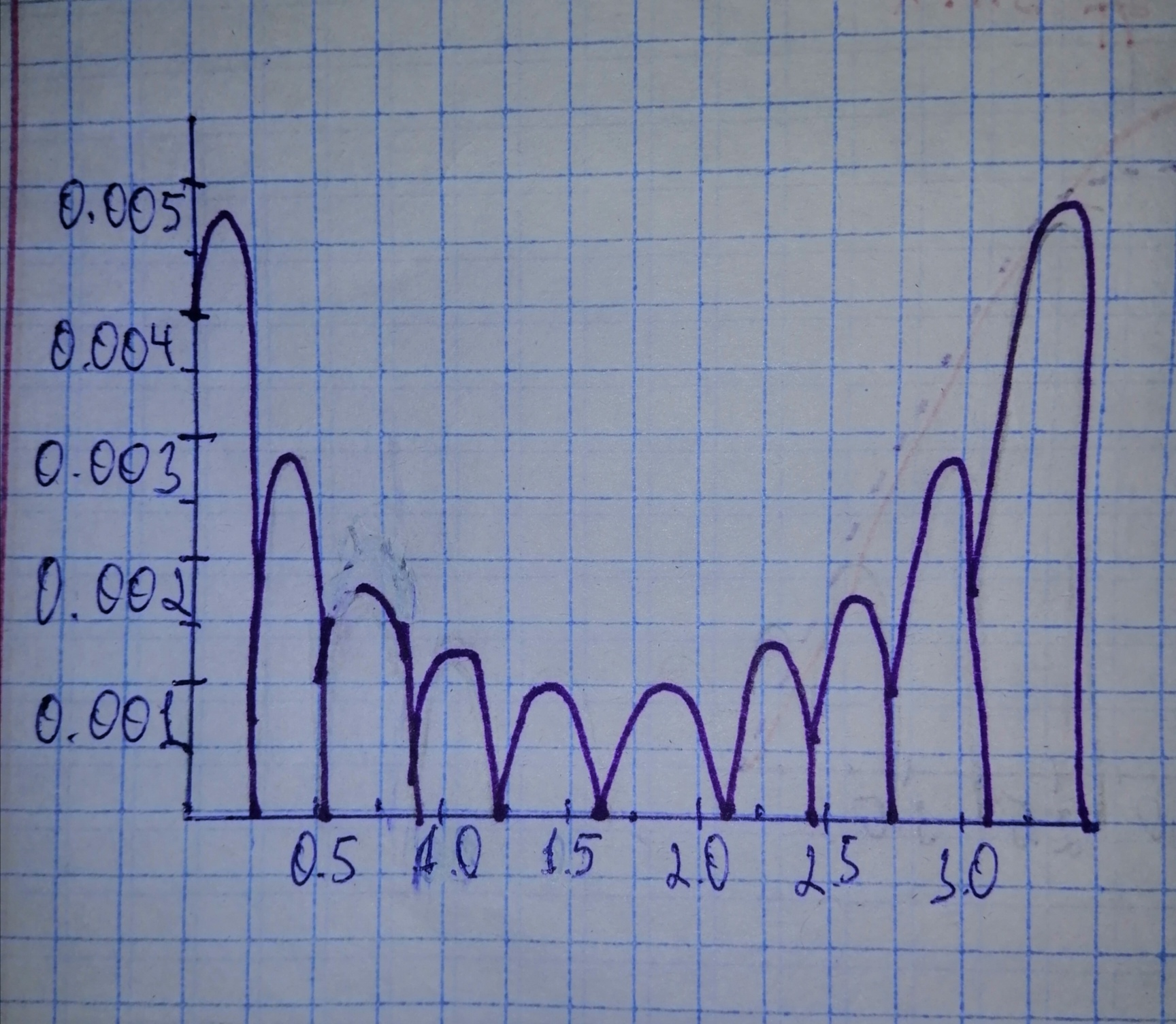# Let S_{N}(x)=frac{4}{pi} sum_{n=1}^{N} frac{1 - (-1)^{n}}{n^{3}} sin(nx). Construct graphs of S_{N}(x) and x(pi - x), for 0 leq x leq pi, for N=2 andDofotheroU 2021-01-05 Answered
Let
Construct graphs of
This will give some sense of the correctness of Fourier’s claim that this polynomial could be exactly represented by the infinite series
You can still ask an expert for help

• Questions are typically answered in as fast as 30 minutes

Solve your problem for the price of one coffee

• Math expert for every subject
• Pay only if we can solve itLayton

Step 1
Note that for even n the coefficient in the sum is zero, so some terms are absent.Step 2Step 3
We see that ${S}_{10}\left(x\right)$ is a very good a approximation of the function

The absolute value of their difference is given below for the comparison: